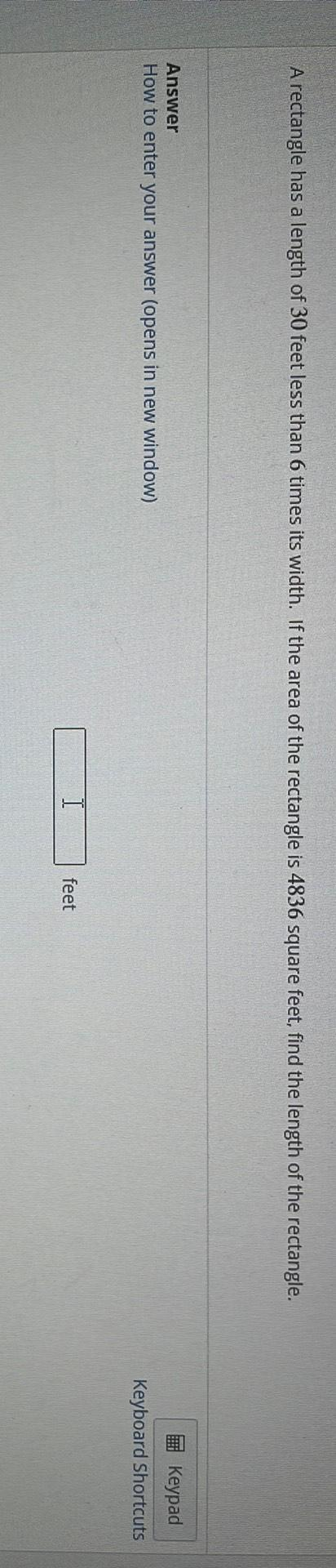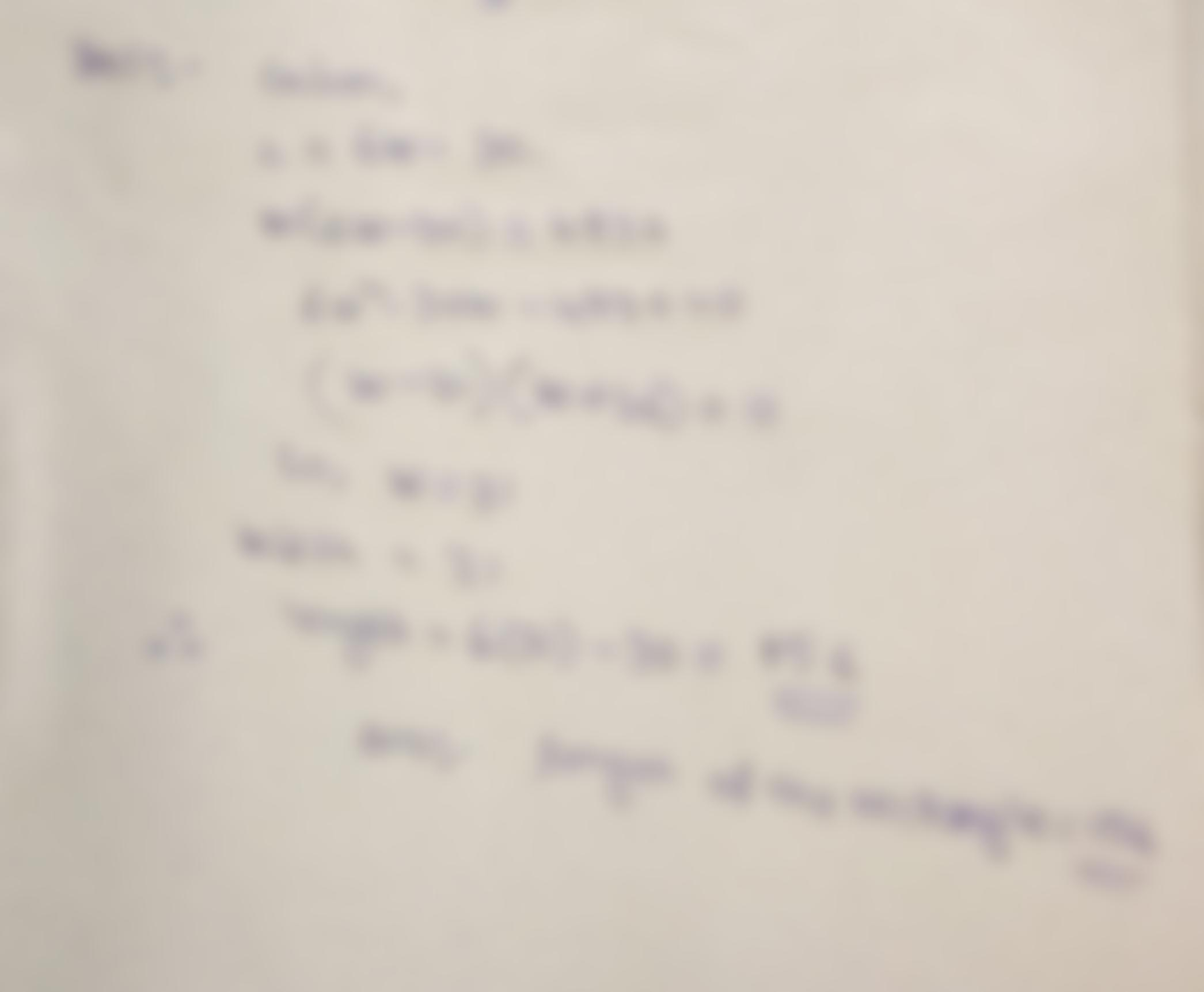Question:

A rectangle has a length of 30 feet less than 6 times its width. If the area of the rectangle is 4836 square feet, find the lengA rectangle has a length of 30 feet less than 6 times its width. If the area of the rectangle is 4836 square feet, find the length of the rectangle. Answer How to enter your answer (opens in new window) Keypad Keyboard Shortcuts feet